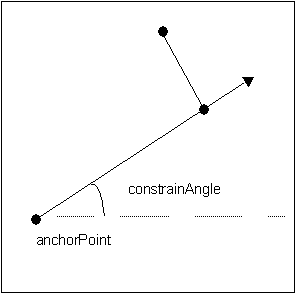This document is archived and information here might be outdated.  Recommended version.

IPoint.ConstrainAngle Method (ArcObjects .NET 10.8 SDK)
 ArcObjects Help for .NET developers > ArcObjects Help for .NET developers > ArcObjects namespaces > Geometry > ESRI.ArcGIS.Geometry > Interfaces > IP > IPoint Interface > IPoint.ConstrainAngle Method
 ArcGIS Developer Help

# IPoint.ConstrainAngle Method

Projects this point to the point on the infinite line defined by anchor and angle (in radians). If allowOpposite is true, then the point can also snap to angle + pi radians.

```[Visual Basic .NET]
Public Sub ConstrainAngle ( _
ByVal constraintAngle As Double, _
ByVal anchor As IPoint, _
ByVal allowOpposite As Boolean _
)
```
```[C#]
public void ConstrainAngle (
double constraintAngle,
IPoint anchor,
bool allowOpposite
);
```
```[C++]
HRESULT ConstrainAngle(
double constraintAngle,
IPoint* anchor,
VARIANT_BOOL allowOpposite
);
```
```[C++]
Parameters
constraintAngle
constraintAngle is a parameter of type double
anchor
anchor is a parameter of type IPoint*
allowOpposite
allowOpposite is a parameter of type bool
```

#### Product Availability

Available with ArcGIS Engine, ArcGIS Desktop, and ArcGIS Server.

#### Description

Projects the base Point to to the nearest point on the line defined by an input anchor point and input angle.  ConstrainAngle is used by the editor to force a newly created Point to be on the line between a fixed point and a specified angle.

#### Remarks

ContrainAngle[C#]

//Finds the closes point to line from (0,0) with angles

//defined by steps of pi/4 (Note all angles in radians)

private void ConstrainAngle()
{
IPoint point = new PointClass();
point.PutCoords(0, 0);

IPoint newPoint = new PointClass();
newPoint.PutCoords(1,0);

for (int i = 0; i < 8; i++)
{
newPoint.ConstrainAngle(i * Math.PI / 4, point, true);

System.Windows.Forms.MessageBox.Show(newPoint.X + ", " + newPoint.Y);
}
}

[Visual Basic .NET]

'Finds the closes point to line from (0,0) with angles
'defined by steps of pi/4 (Note all angles in radians)
'
Sub TestConstrainAngle()
Dim pApoint As ESRI.ArcGIS.Geometry.IPoint
Dim pNpoint As ESRI.ArcGIS.Geometry.IPoint
Dim pi As Double
Dim dAngle As Double
Dim i As Long

pApoint = New ESRI.ArcGIS.Geometry.Point
pi = 4 * Math.Atan(1)
dAngle = 0
pApoint.PutCoords(0, 0)

pNpoint = New ESRI.ArcGIS.Geometry.Point

For i = 0 To 7
pNpoint.PutCoords(1, 0)
dAngle = i * pi / 4
pNpoint.ConstrainAngle(dAngle, pApoint, True)
MsgBox("angle = " & i & "*pi/4" & vbCrLf & pNpoint.X & "," & pNpoint.Y)
Next i
End Sub